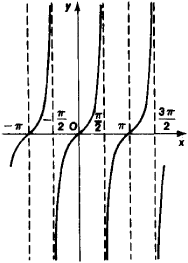# Tangent Curve

The following article is from The Great Soviet Encyclopedia (1979). It might be outdated or ideologically biased.

## Tangent Curve

a plane curve that is the graph of the equation y = tan x, where x is an angle. The tangent curve consists of an infinite number of separate congruent curves that can be obtainedFigure 1. Graph of the equation y = tan x

from each other through a displacement along the x-axis by a distance that is a multiple of π (see Figure 1). The tangent curve intersects the x-axis at the points ± πn (or ±180°n), where n = 0, 1, 2,....